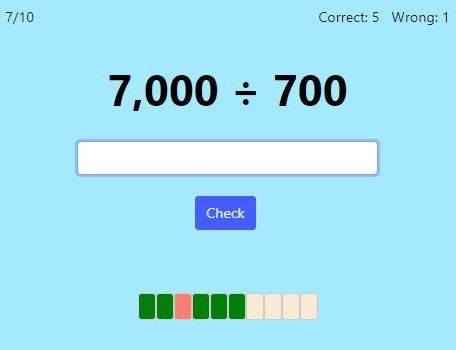MM Practice
×
Multiplication
Division
Place Value
Fractions & Decimals
Measurement
Statistics & Probability
Pre-Algebra
Money
Kindergarten
Geometry

# Divide Whole Numbers Ending in ZerosOnline Practice for grades 4-6

Here, we are not dividing anything BY zero, but practicing mental division problems with numbers that end in zeros, such as 350 ÷ 5, 2,400 ÷ 60, or 540,000 ÷ 6,000.

Students usually start learning this topic in 4th grade, but 5th and 6th grade students usually benefit from practicing it too.

The main principle for solving these division problems is this. Each division is actually built from a simple division fact, such as 54 ÷ 6 or 18 ÷ 3. You will need to solve that problem.

Then think how may zeros must be tagged onto the answer, based on multiplication.

For example, to solve 2,400 ÷ 30, first divide 24 ÷ 3 = 8. Then, think whether the answer would be 8, 80, or 800, based on multiplying each of the 8 × 30, 80 × 300, and 800 × 30, and checking which one will give you the dividend, 2,400.

Another principle that helps in solving these is that you can cancel out the same amount of zeros in each of the dividend and divisor in order to get a new, simpler problem with the same answer as the original.

You can also practice the opposite operation, multiplication with zeros here.

You can choose the level of difficulty, timed or untimed practice, and the number of practice problems.

Screenshot:OR
Practice for a set time: min

Allow my comment to be posted on this site